## 《Machine Learning in Action》—— 剖析支持向量机，单手狂撕线性SVM

SVM内部的数学公式很多，但请不要未战先怯，犯下兵家大忌。无论是阅读该篇文章也好，学习其他相关SVM资源也罢，还请诸君耐心、认真且完整的看完。
SVM的原理过程会涉及到很多的符号或记号，一定要梳理清楚他们所代表的含义。另外，推导过程中会存在很多的向量或矩阵，一定要明白其中shape，这一点可能在不同的资料中会有不一样的处理方式。

## 符号说明表示单个样本中的某个属性特征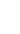表示单个样本所对应的标签（具体分类），为整数，非1即-1表示的是权值行向量，其中，也是所需要训练的参数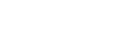表示拉格朗日乘子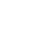在这篇文章中表示线性核函数

## 一、SVM是什幺

Support Vector Machines explained well（可能需要翻墙）： http://bytesizebio.net/2014/02/05/support-vector-machines-explained-well/## 二、线性可分SVM与间隔最大化

import numpy as np
import pylab as pl
from sklearn import svm
%matplotlib inline
print(np.__version__)
"""
Author: Taoye
微信公众号：玩世不恭的Coder
"""
if __name__ == "__main__":
# np.random.seed(100)        # 可自行设置随机种子每次随机产生相同的数据
X_data = np.concatenate((np.add(np.random.randn(20, 2), [3, 3]),
np.subtract(np.random.randn(20, 2), [3, 3])),
axis = 0)      # random随机生成数据，+ -3达到不同类别数据分隔的目的
Y_label = np.concatenate((np.zeros(), np.ones()), axis = 0)
svc = svm.SVC(kernel = "linear")
svc.fit(X_data, Y_label)
w = svc.coef_
ww = -w / w                # 获取权重w
xx = np.linspace(-6, 6)
yy = ww * xx - (svc.intercept_) / w   # intercept_获取结截距
b_down = svc.support_vectors_         # 得到对应的支持向量
yy_down = ww * xx + (b_down - ww * b_down)
b_up = svc.support_vectors_[-1]
yy_up = ww * xx + (b_up - ww * b_up)
pl.plot(xx, yy, "k-"); pl.plot(xx, yy_down, "k--"); pl.plot(xx, yy_up, "k--")
pl.scatter(X_data[:, 0], X_data[:, 1], c = Y_label, cmap = pl.cm.Paired)
pl.show()#### 关于上述提到的一些关于SVM的名词概念，在正式推演之前，还是有必要理解清楚的。不能识别此Latex公式:
\left(
\begin{matrix}
w_1, w_2 \\
\end{matrix}
\right)
\times
\left(
\begin{matrix}
x^{(1)} \\
x^{(2)}
\end{matrix}
\right) \
+b=0 \tag{2-1}，则上述指向方程最终可以表示为: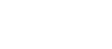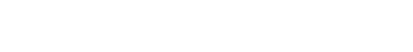#### 例子来源：李航-《统计学习方法》第七章内容## 三、拉格朗日乘数法和对偶问题

#### 例子来源：李亿民-《微积分方法》第二章内容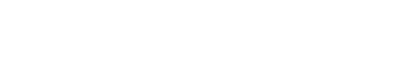，原始目标函数即可转化为：1. 先求内层关于1. 的最小值，此时我们应该将 视为常数

1. 再求外层关于 的最大值，由于经过了第一步关于1. 的最小值，这两个参数已经被消掉了，所以第二步只会存在关于 的求解

## 四、模型的求解

### 关于拉格朗日的内层求解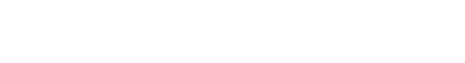#### 例子来源：李航-《统计学习方法》第七章内容求偏导数并令其为0，易知在点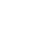，所以最小值应在边界上达到。时，最小值为；当时，最小值。所以，当时，此时达到最小。### 基于SMO算法的外层求解

SMO算法是一种启发式算法，其基本思想是：如果所有变量的解都满足此最优化问题的KKT条件，那幺这个最优化问题的解就得到了。因为KKT条件是该最优化问题的充分必要条件。否则，选择两个变量，固定其他变量，针对这两个变量构建一个二次规划问题。这个二次规划问题关于这两个变量的解就应该更接近原始二次规划问题的解，因为这会使得原始二次规划问题的目标函数值变得更小。更重要的是，这时子问题可以通过解析方法求解，这样就可以大大提高整个算法的计算速度。 子问题有两个变量，一个是违反KKT条件最严重的那一个，另一个由约束条件自动确定。如此，SMO算法将原问题不断分解为子问题并对子问题求解，进而达到求解原问题的目的。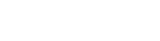PS：关于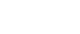是什幺，请见上图中的内容。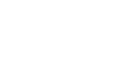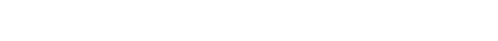## 五、编程手撕线性SVM

"""
Author: Taoye
微信公众号: 玩世不恭的Coder
Explain: 用于生成训练数据集
Parameters:
data_number: 样本数据数目
Return:
x_data: 数据样本的属性矩阵
y_label: 样本属性所对应的标签
"""
def etablish_data(data_number):
x_data = np.concatenate((np.add(np.random.randn(data_number, 2), [3, 3]),
np.subtract(np.random.randn(data_number, 2), [3, 3])),
axis = 0)      # random随机生成数据，+ -3达到不同类别数据分隔的目的
temp_data = np.zeros([data_number])
temp_data.fill(-1)
y_label = np.concatenate((temp_data, np.ones([data_number])), axis = 0)
return x_data, y_label

"""
Author: Taoye
微信公众号: 玩世不恭的Coder
Explain: 随机选取alpha_j
Parameters:
alpha_i_index: 第一个alpha的索引
alpha_number: alpha总数目
Return:
alpha_j_index: 第二个alpha的索引
"""
def random_select_alpha_j(alpha_i_index, alpha_number):
alpha_j_index = alpha_i_index
while alpha_j_index == alpha_i_index:
alpha_j_index = np.random.randint(0, alpha_number)
return alpha_j_index

"""
Author: Taoye
微信公众号: 玩世不恭的Coder
Explain: 使得alpha_j在[L, R]区间之内
Parameters:
alpha_j: 原始alpha_j
L: 左边界值
R: 右边界值
Return:
L,R,alpha_j: 修改之后的alpha_j
"""
def modify_alpha(alpha_j, L, R):
if alpha_j < L: return L
if alpha_j > R: return R
return alpha_j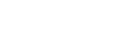"""
Author: Taoye
微信公众号: 玩世不恭的Coder
Explain: 计算误差并返回
"""
def calc_E(alphas, y_lable, x_data, b, i):
f_x_i = float(np.multiply(alphas, y_lable).T * (x_data * x_data[i, :].T)) + b
return f_x_i - float(y_label[i])"""
Author: Taoye
微信公众号: 玩世不恭的Coder
Explain: 计算eta并返回
"""
def calc_eta(x_data, i, j):
eta = 2.0 * x_data[i, :] * x_data[j, :].T \
- x_data[i, :] * x_data[i, :].T \
- x_data[j, :] * x_data[j,:].T
return eta
"""
Author: Taoye
微信公众号: 玩世不恭的Coder
Explain: 计算b1, b2并返回
"""
def calc_b(b, x_data, y_label, alphas, alpha_i_old, alpha_j_old, E_i, E_j, i, j):
b1 = b - E_i \
- y_label[i] * (alphas[i] - alpha_i_old) * x_data[i, :] * x_data[i, :].T \
- y_label[j] * (alphas[j] - alpha_j_old) * x_data[i, :] * x_data[j, :].T
b2 = b - E_j \
- y_label[i] * (alphas[i] - alpha_i_old) * x_data[i, :] * x_data[j, :].T \
- y_label[j] * (alphas[j] - alpha_j_old) * x_data[j, :] * x_data[j, :].T
return b1, b2

OK，准备工作已经完成了，接下来是时候放出我们的核心SMO算法的代码了，大家可根据前面的SMO思想来理解，下面代码也会放出详细的注释来帮助大家理解：

"""
Author: Taoye
微信公众号: 玩世不恭的Coder
Explain: SMO核心算法，求得b和laphas向量
Parameters:
x_data: 样本属性特征矩阵
y_label: 属性特征对应的标签
C：惩罚参数
toler：容错率
max_iter：迭代次数
Return:
b: 决策面的参数b
alphas：获取决策面参数w所需要的alphas
"""
def linear_smo(x_data, y_label, C, toler, max_iter):
x_data = np.mat(x_data); y_label = np.mat(y_label).T     # 将数据转换为矩阵类型
m, n = x_data.shape                                      # 得到数据样本的shape信息
b, alphas, iter_num = 0, np.mat(np.zeros((m, 1))), 0     # 初始化参数b和alphas和迭代次数
while (iter_num < max_iter):                            # 最多迭代max_iter次
alpha_optimization_num = 0   # 定义优化次数
for i in range(m):          # 遍历每个样本，一次选取一个样本计算误差
E_i = calc_E(alphas, y_label, x_data, b, i)      # 样本i的误差计算
if ((y_label[i] * E_i < -toler) and (alphas[i] < C)) or ((y_label[i] * E_i > toler) and (alphas[i] > 0)):
j = random_select_alpha_j(i, m)              # 随机选取一个不与i重复j
E_j = calc_E(alphas, y_label, x_data, b, j)  # 计算样本j的误差
alpha_i_old = alphas[i].copy(); alpha_j_old = alphas[j].copy();
if (y_label[i] != y_label[j]):               # 重新规范alphas的左右区间
L, R = max(0, alphas[j] - alphas[i]), min(C, C + alphas[j] - alphas[i])
else:
L, R = max(0, alphas[j] + alphas[i] - C), min(C, alphas[j] + alphas[i])
if L == R: print("L==R"); continue          # L==R时选取下一个样本
eta = calc_eta(x_data, i, j)                  # 计算eta值
if eta >= 0: print("eta>=0"); continue
alphas[j] -= y_label[j] * (E_i - E_j) / eta
alphas[j] = modify_alpha(alphas[j], L, R)     # 修改alpha[j]
if (abs(alphas[j] - alpha_j_old) < 0.00001): print("alpha_j更改太小"); continue
alphas[i] += y_label[j] * y_label[i] * (alpha_j_old - alphas[j])    # 修改alphas[i]
b1, b2= calc_b(b, x_data, y_label, alphas, alpha_i_old, alpha_j_old, E_i, E_j, i, j)    # 计算b值
if (0 < alphas[i]) and (C > alphas[i]): b = b1
elif (0 < alphas[j]) and (C > alphas[j]): b = b2
else: b = (b1 + b2)/2.0
alpha_optimization_num += 1
print("迭代次数:%d，样本:%d，alphas向量的优化次数:%d" % (iter_num, i+1, alpha_optimization_num))
if (alpha_optimization_num == 0): iter_num += 1
else: iter_num = 0
print("迭代次数:%d" % iter_num)
return b, alphas"""
Author: Taoye
微信公众号: 玩世不恭的Coder
Explain: 根据公式计算出w权值向量
Parameters:
x_data: 样本属性特征矩阵
y_label: 属性特征对应的标签
alphas：linear_smo方法所返回的alphas向量
Return:
w: 决策面的参数w
"""
def calc_w(x_data, y_label, alphas):
x_data, y_label, alphas = np.array(x_data), np.array(y_label), np.array(alphas)
return np.dot((np.tile(y_label.reshape(1, -1).T, (1, 2)) * x_data).T, alphas).tolist()

import pylab as pl
"""
Author: Taoye
微信公众号: 玩世不恭的Coder
Explain: 绘制出分类结果
Parameters:
x_data: 样本属性特征矩阵
y_label: 属性特征对应的标签
w：决策面的w参数
b：决策面的参数b
"""
def plot_result(x_data, y_label, w, b):
data_number, _ = x_data.shape; middle = int(data_number / 2)
plt.scatter(x_data[:, 0], x_data[:, 1], c = y_label, cmap = pl.cm.Paired)
x1, x2 = np.max(x_data), np.min(x_data)
w1, w2 = w, w
y1, y2 = (-b - w1 * x1) / w2, (-b - w1 * x2) / w2
plt.plot([float(x1), float(x2)], [float(y1), float(y2)])    # 绘制决策面
for index, alpha in enumerate(alphas):
if alpha > 0:
b_temp = - w1 * x_data[index] - w2 * x_data[index]
y1_temp, y2_temp = (-b_temp - w1 * x1) / w2, (-b_temp - w1 * x2) / w2
plt.plot([float(x1), float(x2)], [float(y1_temp), float(y2_temp)], "k--")    # 绘制支持向量
plt.scatter(x_data[index], x_data[index], s=150, c='none', alpha=0.7, linewidth=2, edgecolor='red')   # 圈出支持向量
plt.show()
if __name__ == "__main__":
x_data, y_label = etablish_data(50)
b, alphas = linear_smo(x_data, y_label, 0.8, 0.0001, 40)
w = calc_w(x_data, y_label, alphas)
plot_result(x_data, y_label, w, b)#### 完整代码：

import numpy as np
import pylab as pl
from matplotlib import pyplot as plt
class TearLinearSVM:
def __init__(self):
pass
"""
Author: Taoye
微信公众号: 玩世不恭的Coder
Explain: 用于生成训练数据集
Parameters:
data_number: 样本数据数目
Return:
x_data: 数据样本的属性矩阵
y_label: 样本属性所对应的标签
"""
def etablish_data(self, data_number):
x_data = np.concatenate((np.add(np.random.randn(data_number, 2), [3, 3]),
np.subtract(np.random.randn(data_number, 2), [3, 3])),
axis = 0)      # random随机生成数据，+ -3达到不同类别数据分隔的目的
temp_data = np.zeros([data_number])
temp_data.fill(-1)
y_label = np.concatenate((temp_data, np.ones([data_number])), axis = 0)
return x_data, y_label
"""
Author: Taoye
微信公众号: 玩世不恭的Coder
Explain: 随机选取alpha_j
Parameters:
alpha_i_index: 第一个alpha的索引
alpha_number: alpha总数目
Return:
alpha_j_index: 第二个alpha的索引
"""
def random_select_alpha_j(self, alpha_i_index, alpha_number):
alpha_j_index = alpha_i_index
while alpha_j_index == alpha_i_index:
alpha_j_index = np.random.randint(0, alpha_number)
return alpha_j_index
"""
Author: Taoye
微信公众号: 玩世不恭的Coder
Explain: 使得alpha_j在[L, R]区间之内
Parameters:
alpha_j: 原始alpha_j
L: 左边界值
R: 右边界值
Return:
L,R,alpha_j: 修改之后的alpha_j
"""
def modify_alpha(self, alpha_j, L, R):
if alpha_j < L: return L
if alpha_j > R: return R
return alpha_j
"""
Author: Taoye
微信公众号: 玩世不恭的Coder
Explain: 计算误差并返回
"""
def calc_E(self, alphas, y_lable, x_data, b, i):
f_x_i = float(np.dot(np.multiply(alphas, y_lable).T, x_data * x_data[i, :].T)) + b
return f_x_i - float(y_label[i])
"""
Author: Taoye
微信公众号: 玩世不恭的Coder
Explain: 计算eta并返回
"""
def calc_eta(self, x_data, i, j):
eta = 2.0 * x_data[i, :] * x_data[j, :].T \
- x_data[i, :] * x_data[i, :].T \
- x_data[j, :] * x_data[j,:].T
return eta
"""
Author: Taoye
微信公众号: 玩世不恭的Coder
Explain: 计算b1, b2并返回
"""
def calc_b(self, b, x_data, y_label, alphas, alpha_i_old, alpha_j_old, E_i, E_j, i, j):
b1 = b - E_i \
- y_label[i] * (alphas[i] - alpha_i_old) * x_data[i, :] * x_data[i, :].T \
- y_label[j] * (alphas[j] - alpha_j_old) * x_data[i, :] * x_data[j, :].T
b2 = b - E_j \
- y_label[i] * (alphas[i] - alpha_i_old) * x_data[i, :] * x_data[j, :].T \
- y_label[j] * (alphas[j] - alpha_j_old) * x_data[j, :] * x_data[j, :].T
return b1, b2
"""
Author: Taoye
微信公众号: 玩世不恭的Coder
Explain: SMO核心算法，求得b和laphas向量
Parameters:
x_data: 样本属性特征矩阵
y_label: 属性特征对应的标签
C：惩罚参数
toler：容错率
max_iter：迭代次数
Return:
b: 决策面的参数b
alphas：获取决策面参数w所需要的alphas
"""
def linear_smo(self, x_data, y_label, C, toler, max_iter):
x_data = np.mat(x_data); y_label = np.mat(y_label).T     # 将数据转换为矩阵类型
m, n = x_data.shape                                      # 得到数据样本的shape信息
b, alphas, iter_num = 0, np.mat(np.zeros((m, 1))), 0     # 初始化参数b和alphas和迭代次数
while (iter_num < max_iter):                            # 最多迭代max_iter次
alpha_optimization_num = 0   # 定义优化次数
for i in range(m):          # 遍历每个样本，一次选取一个样本计算误差
E_i = self.calc_E(alphas, y_label, x_data, b, i)      # 样本i的误差计算
if ((y_label[i] * E_i < -toler) and (alphas[i] < C)) or ((y_label[i] * E_i > toler) and (alphas[i] > 0)):
j = self.random_select_alpha_j(i, m)              # 随机选取一个不与i重复j
E_j = self.calc_E(alphas, y_label, x_data, b, j)  # 计算样本j的误差
alpha_i_old = alphas[i].copy(); alpha_j_old = alphas[j].copy();
if (y_label[i] != y_label[j]):               # 重新规范alphas的左右区间
L, R = max(0, alphas[j] - alphas[i]), min(C, C + alphas[j] - alphas[i])
else:
L, R = max(0, alphas[j] + alphas[i] - C), min(C, alphas[j] + alphas[i])
if L == R: print("L==R"); continue          # L==R时选取下一个样本
eta = self.calc_eta(x_data, i, j)                  # 计算eta值
if eta >= 0: print("eta>=0"); continue
alphas[j] -= y_label[j] * (E_i - E_j) / eta
alphas[j] = self.modify_alpha(alphas[j], L, R)     # 修改alpha[j]
if (abs(alphas[j] - alpha_j_old) < 0.00001): print("alpha_j更改太小"); continue
alphas[i] += y_label[j] * y_label[i] * (alpha_j_old - alphas[j])    # 修改alphas[i]
b1, b2= self.calc_b(b, x_data, y_label, alphas, alpha_i_old, alpha_j_old, E_i, E_j, i, j)    # 计算b值
if (0 < alphas[i]) and (C > alphas[i]): b = b1
elif (0 < alphas[j]) and (C > alphas[j]): b = b2
else: b = (b1 + b2)/2.0
alpha_optimization_num += 1
print("迭代次数:%d，样本:%d，alphas向量的优化次数:%d" % (iter_num, i+1, alpha_optimization_num))
if (alpha_optimization_num == 0): iter_num += 1
else: iter_num = 0
print("迭代次数:%d" % iter_num)
return b, alphas
"""
Author: Taoye
微信公众号: 玩世不恭的Coder
Explain: 根据公式计算出w权值向量
Parameters:
x_data: 样本属性特征矩阵
y_label: 属性特征对应的标签
alphas：linear_smo方法所返回的alphas向量
Return:
w: 决策面的参数w
"""
def calc_w(self, x_data, y_label, alphas):
x_data, y_label, alphas = np.array(x_data), np.array(y_label), np.array(alphas)
return np.dot((np.tile(y_label.reshape(1, -1).T, (1, 2)) * x_data).T, alphas).tolist()
"""
Author: Taoye
微信公众号: 玩世不恭的Coder
Explain: 绘制出分类结果
Parameters:
x_data: 样本属性特征矩阵
y_label: 属性特征对应的标签
w：决策面的w参数
b：决策面的参数b
"""
def plot_result(self, x_data, y_label, w, b):
data_number, _ = x_data.shape; middle = int(data_number / 2)
plt.scatter(x_data[:, 0], x_data[:, 1], c = y_label, cmap = pl.cm.Paired)
x1, x2 = np.max(x_data), np.min(x_data)
w1, w2 = w, w
y1, y2 = (-b - w1 * x1) / w2, (-b - w1 * x2) / w2
plt.plot([float(x1), float(x2)], [float(y1), float(y2)])    # 绘制决策面
for index, alpha in enumerate(alphas):
if alpha > 0:
b_temp = - w1 * x_data[index] - w2 * x_data[index]
y1_temp, y2_temp = (-b_temp - w1 * x1) / w2, (-b_temp - w1 * x2) / w2
plt.plot([float(x1), float(x2)], [float(y1_temp), float(y2_temp)], "k--")    # 绘制支持向量
plt.scatter(x_data[index], x_data[index], s=150, c='none', alpha=0.7, linewidth=2, edgecolor='red')   # 圈出支持向量
plt.show()
if __name__ == '__main__':
linear_svm = TearLinearSVM()
x_data, y_label = linear_svm.etablish_data(50)
b, alphas = linear_svm.linear_smo(x_data, y_label, 0.8, 0.0001, 40)
w = linear_svm.calc_w(x_data, y_label, alphas)
linear_svm.plot_result(x_data, y_label, w, b)

SVM是学习机器学习必然接触到的一个重要算法，所以一定要对其内在原理了解清楚，并不是说一定要手撕SVM的完整代码，但最起码使用框架的时候要了解内部都做了什幺“小动作”，不要为了用而用。

#### 参考资料：

 《机器学习实战》：Peter Harrington 人民邮电出版社  《统计学习方法》：李航 第二版 清华大学出版社  《机器学习》：周志华 清华大学出版社  《微积分方法》：李亿民 中国海洋出版社

 Support Vector Machines explained well（翻墙）： http://bytesizebio.net/2014/02/05/support-vector-machines-explained-well/